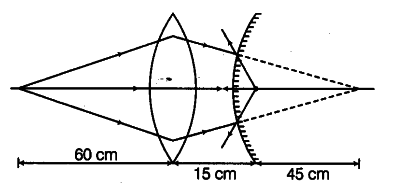# A convex lens of focal length 30 cm is placed coaxially with a convex mirror of radius of curvature 20 cm

A convex lens of focal length 30 cm is placed coaxially with a convex mirror of radius of curvature 20 cm. The two are kept at 15 cm from each other. A point object lies 60 cm in front of the convex lens. Draw a ray diagram to show the formation of the image by the combination. Determine the nature and position of the image formed.

The ray diagram showing the image formation is given below:O is at 2f of lens, so it will form image at 2f on other side, i.e. 60 cm from lens. Hence, position of object from mirror is at (60 -15) cm = 45 cm behind the mirror.

For mirror,

f = +10 cm,
u = +45 cm,
v = ?

As 1/v + 1/u = 1/f
===> 1/v + 1/45 = 1/10

v = +90/7 cm